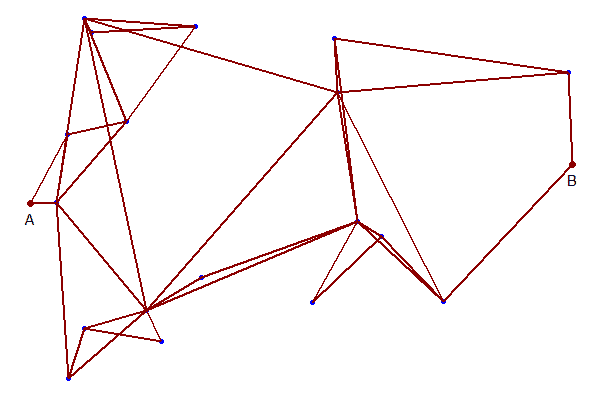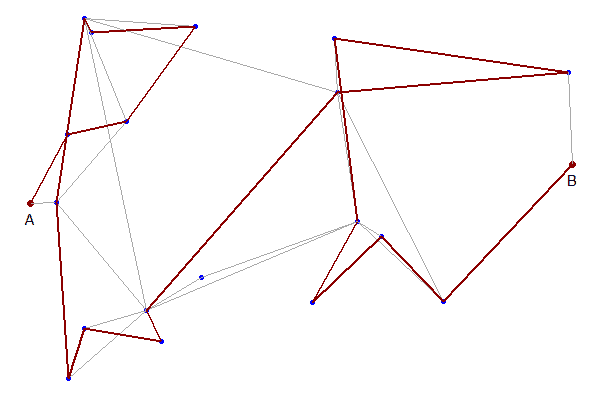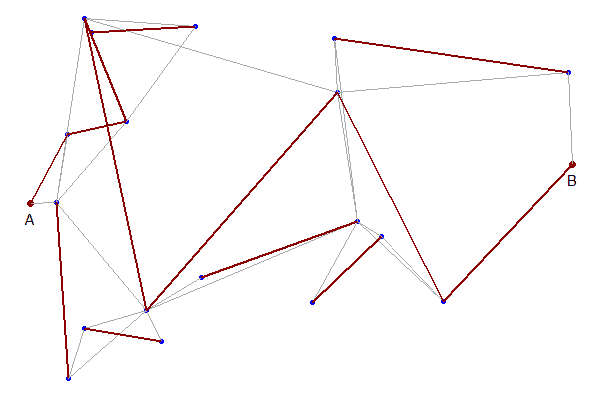## Monday, February 10, 2020

### Longest path problem

This is a question that regularly pops up: how to solve the longest path problem? At first sight, this is easy. Just replace the "minimize" in the shortest path problem by "maximize" and we are good to go. Unfortunately, opposed to the well-known shortest path problem, the longest path problem is not that easy to state and to solve.

#### 1. Standard Shortest Path Formulation

The standard shortest path can be formulated as a Mixed Integer Programming problem:

Shortest Path Problem
\begin{align} \min& \sum_{(i,j)\in\color{darkblue} A} \color{darkblue}d_{i,j} \color{darkred}x_{i,j} \\ & \sum_{j|(j,i)\in \color{darkblue}A} \color{darkred}x_{j,i} + \color{darkblue}b_i = \sum_{j|(i,j)\in \color{darkblue}A} \color{darkred}x_{i,j} && \forall i \\ &\color{darkred}x_{i,j} \in \{0,1\} \\ & \color{darkblue} b_i = \begin{cases}1 & \text{if i is a source node} \\ -1 & \text{if i is a sink node} \\ 0 & \text{otherwise} \end{cases} \end{align}

where $$A$$ is our network and $$b$$ is a sparse data vector indicating the exogenous inflow or outflow at the source or sink node.Network with the shortest path

There are some implicit assumptions in this model: there are no negative cycles. In the above example, $$d_{i,j}$$ represents distance, so we have $$d_{i,j}\ge 0$$ and there is no problem of negative cycles.

Shortest path problems are very easy MIPs to solve. The solution is automatically integer, so it can be solved as an LP and these LPs are very sparse (only two non-zero elements per column) and very easy to solve. In addition, specialized network solvers are widely available.

The problem in the picture has 20 nodes and 37 × 2 arcs. In this example, the arcs are duplicated to make sure we can go in two directions. This leads to an LP of 74 variables and 20 constraints. The solution looks like:

----     75 VARIABLE z.L                   =      128.538  objective

----     75 VARIABLE x.L  flow

point6(B)     point15     point16     point18     point20

point5(A)                               1.000
point15         1.000
point16                                             1.000
point18                                                         1.000
point20                     1.000


#### 2. Just use max

When we just turn the above problem in a maximization problem, we get results that are difficult to interpret. But they certainly don't form what we would call a path:

----     78 VARIABLE z.L                   =     1638.406  objective

----     78 VARIABLE x.L  flow

point1      point2      point3      point4   point5(A)   point6(B)      point7      point8      point9

point1                                              1.000
point3                                                                                                          1.000
point4          1.000
point5(A)                                                                                           1.000
point6(B)                                                                               1.000
point7                                                                      1.000
point8                                                          1.000
point9                                  1.000
point10                     1.000
point12                                                                                                         1.000
point13                                                                                 1.000
point14                                             1.000                                           1.000
point15                                                                     1.000
point16                                                                                             1.000
point17         1.000                               1.000
point18                                 1.000                                                                   1.000
point19                                                                                 1.000
point20                     1.000

+     point10     point11     point12     point13     point14     point15     point16     point17     point18

point1                                                                                              1.000
point2          1.000
point3                                                                                                          1.000
point4                                                          1.000                               1.000
point5(A)                                                                               1.000
point7                                              1.000
point8                                                          1.000                   1.000
point9                                  1.000                                                                   1.000
point10                                                                     1.000
point11                                                                                                         1.000
point12                                                                                 1.000                   1.000
point14                                                                                 1.000       1.000
point15         1.000
point16                                 1.000                   1.000                               1.000       1.000
point17                                                         1.000                   1.000                   1.000
point18                     1.000       1.000                                                       1.000
point19                                             1.000                   1.000                   1.000       1.000
point20         1.000       1.000                   1.000                   1.000

+     point19     point20

point2                      1.000
point7          1.000
point10                     1.000
point11                     1.000
point13         1.000       1.000
point15         1.000
point17         1.000
point18         1.000       1.000
point19                     1.000
point20         1.000Maximization results

Basically, all but a few lines in the plot are traveled in both directions. Conclusion: this is not what we are looking for.

#### 3. TSP-like solution

To form a proper path (called an elementary path), we need to add two sets of constraints :
1. The outflow of each node goes to just one arc: $\sum_{j|(i,j)\in A} x_{i,j} \le 1\>\forall i$
2. Forbid any sub-tours to be formed. Sub-tour elimination constraints are well-known from the Traveling Salesman Problem.
Using the simple MTZ (Miller, Tucker, and Zemlin) approach, we can formulate:

Longest Path Problem
\begin{align} \max& \sum_{(i,j)\in\color{darkblue} A} \color{darkblue}d_{i,j} \color{darkred}x_{i,j} \\ & \sum_{j|(j,i)\in \color{darkblue}A} \color{darkred}x_{j,i} + \color{darkblue}b_i = \sum_{j|(i,j)\in \color{darkblue}A} \color{darkred}x_{i,j} && \forall i \\ & \sum_{j|(i,j)\in \color{darkblue}A} \color{darkred}x_{i,j} \le 1 && \forall i\\ & \color{darkred}t_j \ge \color{darkred}t_i + 1 + (\color{darkblue}n-1)(\color{darkred}x_{i,j}-1) && \forall i,j|i\ne\text{source},j\ne\text{sink} \\&\color{darkred}x_{i,j} \in \{0,1\} \\ & \color{darkred}t_i \ge 0 \end{align}

Here $$n$$ is the number of nodes.

The results look like:

----    126 VARIABLE z.L                   =      432.987  objective

----    126 VARIABLE x.L  flow

point1      point2      point3      point4   point6(B)      point7      point8      point9     point10     point12

point2                                                                                                          1.000
point4          1.000
point5(A)                                                                               1.000
point9                                  1.000
point12                                                                                             1.000
point14                                             1.000
point15                                                         1.000
point16                                                                                                                     1.000
point19                                                                     1.000
point20                     1.000

+     point13     point14     point15     point16     point17     point18     point19     point20

point1                                                          1.000
point3                                                                      1.000
point7          1.000
point8                      1.000
point10                                 1.000
point13                                                                                             1.000
point17                                             1.000
point18                                                                                 1.000Solution of Longest Elementary Path Model

This looks much better.

More formulations can be found in .

Let's drop the sub-tour elimination constraints, and have a look at the solution of the remaining model:

Problem A: drop sub-tour elimination constraints
\begin{align} \max& \sum_{(i,j)\in\color{darkblue} A} \color{darkblue}d_{i,j} \color{darkred}x_{i,j} \\ & \sum_{j|(j,i)\in \color{darkblue}A} \color{darkred}x_{j,i} + \color{darkblue}b_i = \sum_{j|(i,j)\in \color{darkblue}A} \color{darkred}x_{i,j} && \forall i \\ & \sum_{j|(i,j)\in \color{darkblue}A} \color{darkred}x_{i,j} \le 1 && \forall i\\ &\color{darkred}x_{i,j} \in \{0,1\} \end{align}

When we look at the solution we see a number of sub-tours of just two points. This means: travel from $$C\rightarrow D$$ and back.Sub-tours with 2 points

These special sub-tours are easily prevented: add a cut of the form $x_{i,j}+x_{j,i} \le 1$ We can add them only for the $$i \rightarrow j \rightarrow i$$ offenders or just for all possible $$i \lt j$$. Here I did the last thing. The model becomes:

\begin{align} \max& \sum_{(i,j)\in\color{darkblue} A} \color{darkblue}d_{i,j} \color{darkred}x_{i,j} \\ & \sum_{j|(j,i)\in \color{darkblue}A} \color{darkred}x_{j,i} + \color{darkblue}b_i = \sum_{j|(i,j)\in \color{darkblue}A} \color{darkred}x_{i,j} && \forall i \\ & \sum_{j|(i,j)\in \color{darkblue}A} \color{darkred}x_{i,j} \le 1 && \forall i\\ & \color{darkred}x_{i,j}+\color{darkred}x_{j,i} \le 1 && \forall i \lt j \\ &\color{darkred}x_{i,j} \in \{0,1\} \end{align}

After adding these cuts and solving problem B we see:Results after adding cuts

The solution has no new sub-tours, so we can conclude this is the optimal solution.

Thus far this is a bit of an ad-hoc method. This approach would fail if we observe more complex sub-tours. However, it is not too difficult to make this more general. We can add appropriate cuts when we observe sub-tours and resolve the model. Such a cutting plane algorithm can actually outperform models where we add sub-tour elimination constraints in advance. With modern solvers, it is even possible to add this cut generation inside the branch-and-bound search (i.e. no resolve needed).

#### Conclusion

The conclusion is: "solve the longest path problem" is not as easy as it seems. We need to employ TSP-like machinery to solve this problem. That means no easy MIP models. Straight MIP models are both not that simple to formulate and will only work for relatively small models. A cutting plane approach can help here.

#### References

1. Leonardo Taccari, Integer programming formulations for the elementary shortest path problem, European Journal of Operational Research, Volume 252, Issue 1, 1 July 2016, Pages 122-130